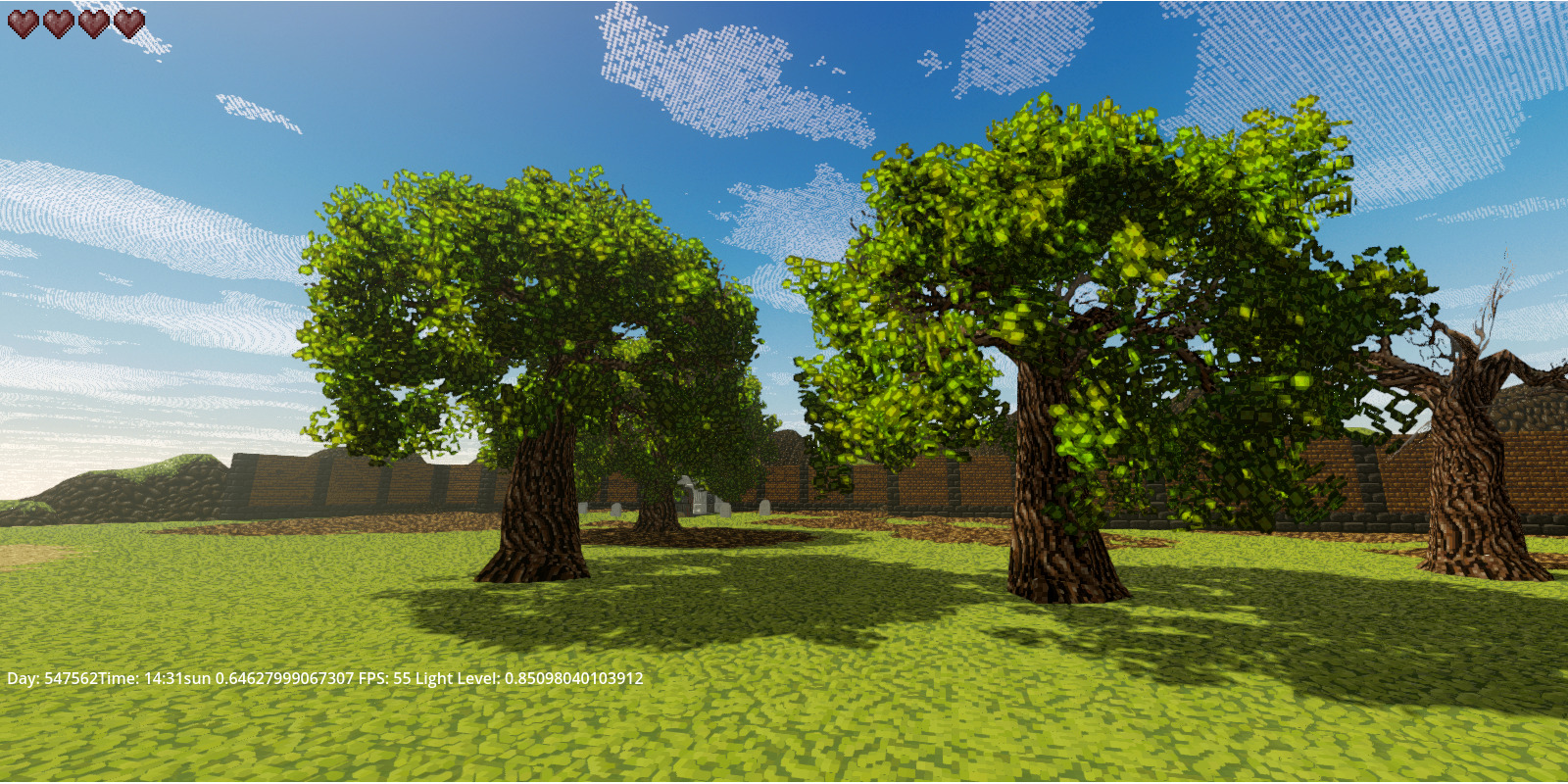# Volumetric Billboards (3D Texture sampled by plane stacking)

Wrote this for a personal project. Please feel free to use it as you see fit, and if possible, kindly provide citations. Your acknowledgment is greatly appreciated!

You will need to apply this shader to geometry with unmerged quads. The higher the number of quads, the greater the resolution (and overdraw too, yikes!).

Additionally, the shader utilizes a 3D texture to sample the volume. You can generate one using MagicaVoxel or any other software of your preference.

I’m using 34 quads per particle cluster in the example images, sampling a 16x16x16 voxels texture. For pixel art style it works fine.

For good results use a 3D model where Quad_num > Texture_width * 2.

Dio from Lunar Blank.

``````shader_type spatial;
render_mode blend_mix, cull_front, depth_prepass_alpha;

uniform float sqrt3 = 1.73205080757;
uniform float particle_size = 2.0;

uniform sampler3D alpha_tex : source_color, filter_nearest;
varying vec4 vertx;

void vertex() {

// we'll use the UV's of each plane in our geometry to billboard them
// center the UV's
vec2 off_uv = UV - vec2(0.5,0.5);
// for each QUAD, offset its vertices
// we are using their IDs to know whether a vertex is part of a QUAD
vec3 new_uv = vec3(off_uv, (-float(VERTEX_ID/4)*(sqrt3/quads_num_x2))) + vec3(0,0,0.5);
// transform into billboards
VERTEX = particle_size * vec4( vec4(new_uv,0.0) * VIEW_MATRIX * MODEL_MATRIX ).xyz;
// pass coordinates to fragment so the 3D texture can be sampled
vertx = 1.0/particle_size * 2.0*sqrt3 * vec4(VERTEX,0.0) * MODEL_MATRIX;
}

void fragment() {

// normals in world space to use for ligthing
vec4 v = (INV_VIEW_MATRIX * vertx) - vec4(NODE_POSITION_WORLD,0.0);
v = v * inverse(MODEL_MATRIX);

// offset our vertices
vec4 newvertx = vertx/2.0 + vec4(0.5,0.5,0.5,0.0);

// make normals point up
v = newvertx * vec4(0,1.0,0,0);

// sample the texture
vec4 tex_sample = texture(alpha_tex, newvertx.xyz);
// blend texture with the position to add color variation
ALBEDO = tex_sample.xyz * 1.4*mix(vec3(0.6,0.4,0.2), newvertx.xyz, 0.2);
// alpha clip the bounding box
if( (newvertx.z < 1.0 && newvertx.z > 0.0) &&
(newvertx.y < 1.0 && newvertx.y > 0.0) &&
(newvertx.x < 1.0 && newvertx.x > 0.0)){

ALPHA = clamp( tex_sample.w, 0.0, 1.0 );
}
else {
ALPHA = 0.0;
}
ALPHA_SCISSOR_THRESHOLD = 0.5;
ROUGHNESS = 0.9;
SPECULAR = 0.2;
NORMAL = normalize(v.xyz);
}

void light() {
// for directional light (sun/moon) apply a yellowish-green to highlight the leaves
if(LIGHT_IS_DIRECTIONAL)
{										//change this color if you are not using for vegetation
DIFFUSE_LIGHT = clamp(ATTENUATION * vec3(0.6,0.7,0.0) * LIGHT_COLOR, 0.0, 1.0);
}
}``````
###### Tags
billboards, particle, Vegetation, Volume, volumetricThe shader code and all code snippets in this post are under CC0 license and can be used freely without the author's permission. Images and videos, and assets depicted in those, do not fall under this license. For more info, see our License terms.

### Volumetric nebulae/clouds

Subscribe
Notify of